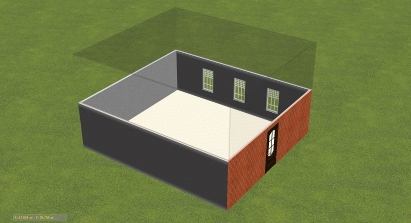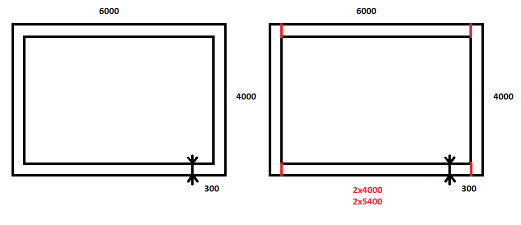homechevron_rightStudychevron_rightMathchevron_rightGeometry

# Total volume

This calculator calculates total volume as sum of volumes of entered rectangular parallelepipeds.

This calculator calculates total volume as sum of volumes of entered rectangular parallelepipeds.

You can see example of many rectangular parallelepipeds in one place on the picture below:That is, to calculate total volume of material, for example, in case of building demolition, you should break down its structure to primitive figures, rectangular parallelepipeds, or cuboids, and enter their dimensions into calculator. For example, there are four walls, floor, ceiling, door and three windows on the picture. To get total volume of material you need to add volumes of walls, floor and ceiling and subtract volumes of door and windows.

You just should be careful when breaking down structure to figures to avoid double counting of same volumes. The picture below illustrates the problem:Here you need to subtract width for two walls to get correct dimensions. Well, it is simple enough and I hope that the idea is clear.#### Volumes

arrow_upwardarrow_downwardLength, mmarrow_upwardarrow_downwardWidth, mmarrow_upwardarrow_downwardHeight, mmarrow_upwardarrow_downwardQuantityarrow_upwardarrow_downwardTypearrow_upwardarrow_downwardHint
Items per page:

Digits after the decimal point: 3